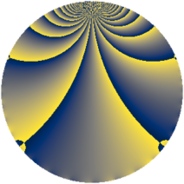# Properties

 Label 1210.2.oLevel $1210$ Weight $2$ Character orbit 1210.o Rep. character $\chi_{1210}(89,\cdot)$ Character field $\Q(\zeta_{22})$ Dimension $660$ Sturm bound $396$

# Related objects

## Defining parameters

 Level: $$N$$ $$=$$ $$1210 = 2 \cdot 5 \cdot 11^{2}$$ Weight: $$k$$ $$=$$ $$2$$ Character orbit: $$[\chi]$$ $$=$$ 1210.o (of order $$22$$ and degree $$10$$) Character conductor: $$\operatorname{cond}(\chi)$$ $$=$$ $$605$$ Character field: $$\Q(\zeta_{22})$$ Sturm bound: $$396$$

## Dimensions

The following table gives the dimensions of various subspaces of $$M_{2}(1210, [\chi])$$.

Total New Old
Modular forms 2020 660 1360
Cusp forms 1940 660 1280
Eisenstein series 80 0 80

## Trace form

 $$660q + 66q^{4} - 4q^{5} + 4q^{6} - 652q^{9} + O(q^{10})$$ $$660q + 66q^{4} - 4q^{5} + 4q^{6} - 652q^{9} + 18q^{10} - 46q^{11} + 4q^{14} + 4q^{15} - 66q^{16} - 16q^{19} + 4q^{20} - 4q^{24} - 4q^{26} - 4q^{29} + 4q^{30} + 8q^{34} + 8q^{35} - 74q^{36} - 16q^{39} + 4q^{40} + 36q^{41} + 2q^{44} + 36q^{45} + 12q^{46} - 50q^{49} - 24q^{50} + 128q^{51} - 16q^{54} + 50q^{55} - 4q^{56} + 4q^{59} - 4q^{60} + 36q^{61} + 66q^{64} - 82q^{65} - 8q^{66} + 16q^{69} + 36q^{70} - 48q^{71} - 8q^{75} + 38q^{76} - 72q^{79} - 4q^{80} + 676q^{81} + 80q^{85} - 8q^{86} + 192q^{89} - 68q^{90} + 92q^{91} + 108q^{94} - 176q^{95} + 4q^{96} + 206q^{99} + O(q^{100})$$

## Decomposition of $$S_{2}^{\mathrm{new}}(1210, [\chi])$$ into newform subspaces

The newforms in this space have not yet been added to the LMFDB.

## Decomposition of $$S_{2}^{\mathrm{old}}(1210, [\chi])$$ into lower level spaces

$$S_{2}^{\mathrm{old}}(1210, [\chi]) \cong$$ $$S_{2}^{\mathrm{new}}(605, [\chi])$$$$^{\oplus 2}$$